# What Is Oscillation Frequency: Interesting Facts and FAQs

Whether our vision can reach them or not, oscillations occur all around us. So, in this post, we’ll look at what is oscillation frequency, how to calculate oscillation frequency and oscillation frequency units.

The frequency of oscillations is a physical term that describes how many oscillations took place in a unit of time in an oscillating system such as a pendulum, spring mass system, or electronic system.

Before we go into what is oscillation frequency, let’s first define oscillation.

## Oscillation:

Oscillation is a repeated variation in amplitude or position around a central point or equilibrium, with time following the same path.

A single oscillation is a complete movement over a period of time, which can be up and down or side to side. One cycle of oscillation is another name for it. The time it takes to complete one oscillation is the oscillation time period. Every oscillation is a periodic motion, but not all periodic motions are oscillations. Examples of oscillation are spring movement with mass, pendulum motion, the vibration of string instruments, etc.Oscillating Pendulum GIF Credits: Wikinana38, ลูกตุ้มธรรมชาติ, CC BY-SA 4.0

## Oscillation frequency:

The oscillation frequency is the number of oscillations that repeat in unit time, i.e., one second. Taking reciprocal of time taken by oscillation will give the frequency of oscillation.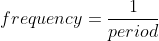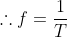To understand oscillation frequency, let’s consider a simple example of the guitar. While playing guitar, you pluck the string of a guitar. As the motion of the guitar string is repetitive for significant cycles, it is considered as oscillation. To complete one successive cycle time taken by the string is the same. Thus, if we take reciprocal of it, we get the frequency of guitar string oscillation. Suppose it takes 0.2 seconds to complete one cycle, then the number of oscillations in one second is five, which is the frequency of oscillating guitar strings.Image Credits: “Guitar strings close up” by richard_north , CC BY 2.0

Let us now look at the frequency of oscillation units.

## Frequency of oscillation units:

The SI unit for the oscillation time period is the second (s). Because the reciprocal of the oscillation’s time period determines its frequency, its unit is the reciprocal of the time period’s unit, i.e., s-1.

Many unit names are inspired by the scientists who worked on the unit’s research. Heinrich Hertz, a German physicist, made a significant contribution to the field of electromagnetic radiation. As a result, Hertz is also regarded as a SI unit of frequency in honour of this famous scientist. As Hertz represents cycles per second, we can say that

1 Hertz = one cycle per second.

Thus, s and Hertz are frequency of oscillation units that are used as SI frequency units, but Hertz is more commonly used.

After going through what is oscillation frequency and frequency oscillation units, you might wonder how to calculate oscillation frequency. So let’s see how to calculate oscillation frequency.

## How to calculate oscillation frequency?

There are two ways to calculate oscillation frequency:

• Mathematical
• Experimental

## ⇒ A mathematical way to calculate oscillation frequency:

To calculate the frequency of an oscillation, we must utilize period and frequency formulas. The formulas for calculating oscillation frequency vary depending on the system.

## ✦ Oscillation frequency of spring mass system:

As shown in the figure, a mass m is attached to the end of the spring. Spring will oscillate up and down due to the weight of the mass. Thus, if k is the spring constant of spring, then the frequency of oscillating frequency is given by: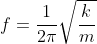## ✦ Oscillation frequency of simple pendulum:

In a simple pendulum, mass m is attached to the end of the string. Thus, due to the mass, the string will oscillate back and forth. Therefore, if L is the length of the string, the frequency of the simple pendulum is given by: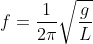GIF credits: Stündle (modification Ideophagous), Pendulum-no-text, CC BY-SA 4.0

Thus, we can say that in the case of a simple pendulum, the frequency of oscillation is not dependent on the mass of the attached object.

## ✦ Oscillation frequency of thecompound or physical pendulum:

A physical or compound pendulum is formed by suspending a rigid body to the fixed horizontal axis. As the rigid body is involved, its moment of inertia I will also come into action. Thus, the frequency of the compound pendulum is given by: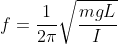## ✦ Oscillation frequencyof the torsional pendulum:

When a disc-like mass is suspended from a wire or thin rod instead of a rigid body, the system is known as a torsional pendulum. As a result, the torsional pendulum oscillation frequency is: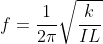## ⇒ An experimental way to calculate oscillation frequency:

The oscillation frequency calculation for a pendulum or a spring mass system is nearly identical. The main difference is that the spring constant k must be calculated for the spring mass system. The method is as follows:

• In a spring mass system, to calculate the spring constant, hang the mass on the spring and the spring will start to oscillate. Now, allow the mass to come to a motionless state where the gravitational force of mass and spring force will be balanced. After that, adding mass to spring will stretch, so record this change in spring stretch.

Δmg -kΔy =0

Thus, spring constant k is: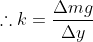Here, Δy is the change in spring stretch.

• Oscillate the spring or pendulum now. Then, for each mass, keep track of the time for ten oscillations. To acquire an accurate response, repeat this method three times. The average of the three times will be used to determine the time period. Take the inverse of that and you’ll get the frequency.

We hope that this article has answered your questions about what oscillation frequency is, what oscillation frequency units are, and how to calculate oscillation.

Alpa P. Rajai

I am Alpa Rajai, Completed my Masters in science with specialization in Physics. I am very enthusiastic about Writing about my understanding towards Advanced science. I assure that my words and methods will help readers to understand their doubts and clear what they are looking for. Apart from Physics, I am a trained Kathak Dancer and also I write my feeling in the form of poetry sometimes. I keep on updating myself in Physics and whatever I understand I simplify the same and keep it straight to the point so that it deliver clearly to the readers. You can also reach me at : https://www.linkedin.com/in/alpa-rajai-858077202/